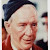# Coordinate transformation for $$\nabla^2$$ operator

Note by , created over 4 years ago

## Converting the $\nabla^2$ operator from 3D cartesian to 3D spherical polar coordinates.9100Created by Domhnall Murphy over 4 years ago
GCSE Maths: Understanding Pythagoras' Theorem
GCSE Maths Symbols, Equations & Formulae
STEM AND LEAF DIAGRAMS
ART NOUVEAU
ACRP Certification Exam Preparation Course - Practice Questions
A level biology Revision I
GCSE Subjects
Forces and motion
Fractions and percentages
New GCSE Maths required formulae

### Page 1

The equation below shows the $$\nabla^2$$ operator in three-dimensional cartesian coordinates$\nabla^2 = \frac{\partial^2}{\partial x^2} + \frac{\partial^2}{\partial y^2} + \frac{\partial^2}{\partial z^2}$